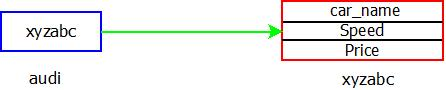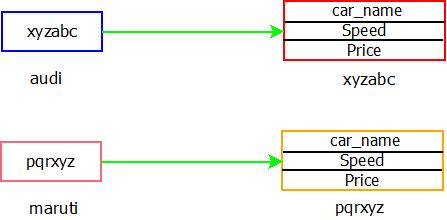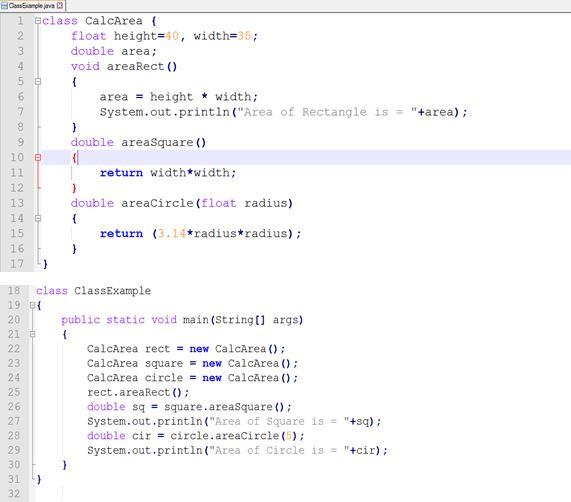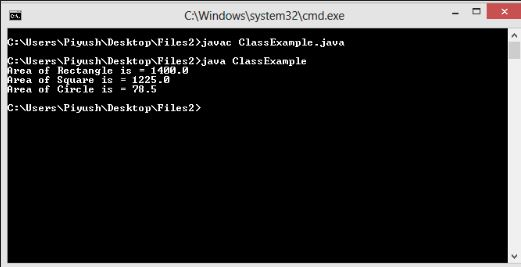# JAVA CLASSES METHODS OBJECTS

Classes, Methods & Objects | Core Java Tutorial | Minigranth

# Classes, Methods and Objects

1. ### Classes In Java : Introduction

• Class is a group of similar type of objects. It was previously defined that class is a blueprint of an object and an object is an instance of a class.
• Now let us learn how to define classes.

•  class class_name {             instance variable1;             instance variable2;             …..             method1() { method body.. }             method2() { method body.. }             ……… }

• The field or variables defined inside a class are called instance variable. Methods within a class exposes the behavior of an object. Instance variables and methods defined inside the class are collectively called members of class.
• Still bothered? Let's look at the example below.

•  class Cars {      String car_name;      int speed;      long price;  }

• The above is an example of how to declare a class. Now we need to create objects of the class to bring class into existence.

2. ### Objects In Java : Introduction

• Now let us learn how to create an object of a class or instance of a class. Let's look at the syntax first and then we will try to create an object through an example.
• Syntax  class_name  ­Object_name = new class_name();

Example
• Lets’s take the above class example and create the objects.
 Cars audi = new Cars();   //object 1
or  Cars audi; audi = new Cars();             Cars maruti = new Cars();  //object 2

• We can declare and define any number of  objects for a class. Lets’s understand the statement " Cars audi = new Cars(); "
Here,
• Cars is the class name.
• audi is the object name
• new is a keyword which is used to dynamically allocate memory for an object and return a reference to it (reference here means address of object in memory) . The memory is allocated in heap area.
• Cars() is the default constructor call. We will see constructors in upcoming section. Now just understand that this statement will initialize the fields of an object.
 Note1 : In the above example, statements Cars audi   &  audi = new Cars() are different. Cars audi ;  // declaring a variable of a class that is object At this point no memory is allocated to an object. It’s simply declares a reference to an object, it contains null at this point. audi = new Cars(); // dynamically allocating memory At this point memory is allocated to an object in heap area using new. Now the reference is assigned to the actual object i.e. audi. When memory is allocated to the object , the reference variable audi will hold the memory address of the actual audi  object i.e. the memory address of heap where object is allocated.Example : Objects In Java

• The above figure shows that audi holds the reference to the actual object in memory, i.e. it’s memory address.
 Note 2 :  Each object defined will have the similar copies of fields. In above example both audi and maruti object will have a copy of variables car_name, speed and price.Example : Objects In Java

#### What happens on assigning one object to other ?

• Let’s take an example,
•  Cars honda = new Cars(); Cars hyundai = honda;

• Now both reference variable honda and hyundai  will refer to same object in memory. Since any changes made using honda will reflect in hyundai too as both refer to same object in memory.
• Hence the conclusion is that on assigning one object reference variable to another object reference variable we are not creating the copy of the object, we are only creating a copy of the reference.

•Example : Objects In Java

#### How will you access a instance variable using objects ?

• By using dot (.) operator.
• Suppose in example of class above we want to set the speed of audi to be 300 and price to be 2000000 then we will write the statement.
•  audi.speed = 300; audi.price = 2000000;

3. ### Methods In Java : Introduction

• As we know that classes contains instance variables and methods, now we will be discussing about methods.
• Recall that we talked about the attributes of an object and one of them was behavior of an object which  is defined by the method .
• Methods are the members of the class that defines or exposes the behavior of an object.
• Methods may or may not take parameters and may or may not return values . Methods having void as a return type do not return a value whereas methods with return type other than void has to return a value. Methods having return type as the class name should return instance of the class.
•

#### How do you invoke methods ?

• Same as we accessed instance variable i.e. by using dot (.) operator.
•  object.method_name();
• Suppose we have a run() method in class Cars that do not return a value the we will write
•  audi.run()

Example
• Let’s take example of another class and create methods in it.Classes, Methods & Objects : ExampleClasses, Methods & Objects : Output

• In the above example we have used three methods of all types with and without parameters and with and without return .